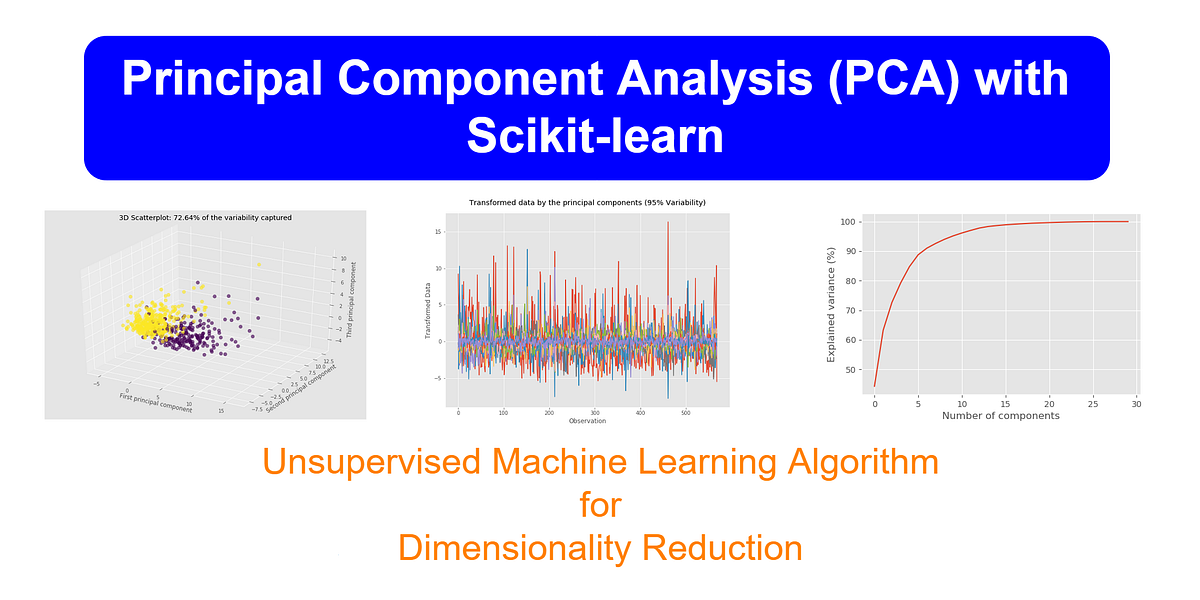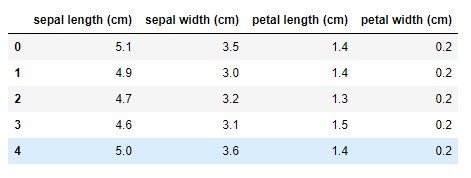# Principal Component Analysis (PCA) with Scikit-learnI’ll explain the dimensionality of a dataset, what dimensionality reduction means, main approaches to dimensionality reduction, reasons for dimensionality reduction and what PCA means. Then, I will go deeper into the topic PCA by implementing the PCA algorithm with Scikit-learn machine learning library.

Hi everyone! This is the second unsupervised machine learning algorithm that I’m discussing here. This time, the topic is Principal Component Analysis (PCA). At the very beginning of the tutorial, I’ll explain the dimensionality of a dataset, what dimensionality reduction means, main approaches to dimensionality reduction, reasons for dimensionality reduction and what PCA means. Then, I will go deeper into the topic PCA by implementing the PCA algorithm with Scikit-learn machine learning library. This will help you to easily apply PCA to a real-world dataset and get results very fast.

In a separate article (not in this one), I will discuss the mathematics behind the principal component analysis by manually executing the algorithm using the powerful numpy and pandas libraries. This will help you to understand how PCA really works behind the scenes.

I highly recommend you to read my previous articles published at Data Science 365before proceeding to read this one. This is because you should have a clear understanding of the basics of numpy, pandas, matplotlib, seaborn and machine learning to understand the codes and concepts discussing here.

## What is dimensionality reduction?

Before we consider reducing the dimensionality of a dataset, we should learn what dimensionality is. Simply, dimensionality is the number of dimensions, features or input variables associated in a dataset. Often, it can be thought as the number of columns (except the label column) in a dataset. The following table shows a part of the iris dataset which contains four features. So, the number of dimensions is four. This means, for example, to demonstrate the first data point in the four-dimensional space, we use p1(5.1, 3.5, 1.4, 0.2) notation.Image by author

Dimensionality reduction means reducing the number of features in a dataset. Dimensionality reduction algorithms project high-dimensional data to a low-dimensional space while retaining as much of the variation (i.e., salient information) as possible.

## Applied Data Analysis in Python Machine Learning and Data Science | Scikit-Learn

Applied Data Analysis in Python Machine learning and Data science, we will investigate the use of scikit-learn for machine learning to discover things about whatever data may come across your desk.

## Dimensionality Reduction in Machine Learning

Dimensionality reduction, or dimension reduction, is the transformation of data from a high-dimensional space into a low-dimensional space so that the low-dimensional representation retains some meaningful properties of the original data.

## Getting Started with scikit-learn Pipelines for Machine Learning

Getting Started with scikit-learn Pipelines for Machine Learning: Building a pipeline from the ground up. (All code in this post is also included in this GitHub repository.)

## Most popular Data Science and Machine Learning courses — July 2020

Most popular Data Science and Machine Learning courses — August 2020. This list was last updated in August 2020 — and will be updated regularly so as to keep it relevant

## 15 Machine Learning and Data Science Project Ideas with Datasets

Learning is a new fun in the field of Machine Learning and Data Science. In this article, we’ll be discussing 15 machine learning and data science projects.